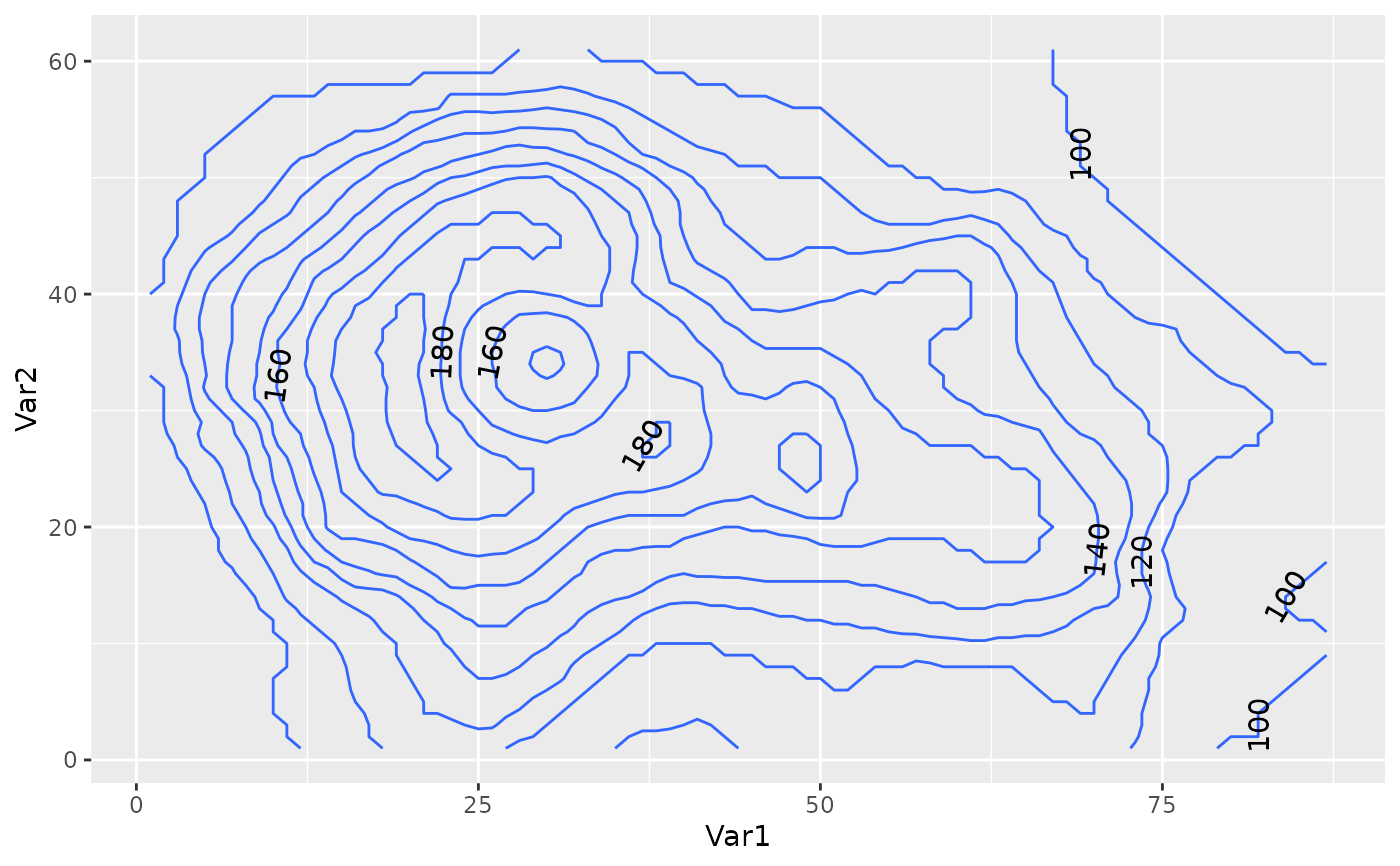Draws labels on contours built with ggplot2::stat_contour.

geom_label_contour(
mapping = NULL,
data = NULL,
stat = "text_contour",
position = "identity",
...,
min.size = 5,
skip = 1,
label.placer = label_placer_flattest(),
parse = FALSE,
nudge_x = 0,
nudge_y = 0,
label.r = grid::unit(0.15, "lines"),
label.size = 0.25,
na.rm = FALSE,
show.legend = NA,
inherit.aes = TRUE
)

geom_text_contour(
mapping = NULL,
data = NULL,
stat = "text_contour",
position = "identity",
...,
min.size = 5,
skip = 1,
rotate = TRUE,
label.placer = label_placer_flattest(),
parse = FALSE,
nudge_x = 0,
nudge_y = 0,
stroke = 0,
check_overlap = FALSE,
na.rm = FALSE,
show.legend = NA,
inherit.aes = TRUE
)

## Arguments

mapping

Set of aesthetic mappings created by aes(). If specified and inherit.aes = TRUE (the default), it is combined with the default mapping at the top level of the plot. You must supply mapping if there is no plot mapping.

data

The data to be displayed in this layer. There are three options:

If NULL, the default, the data is inherited from the plot data as specified in the call to ggplot().

A data.frame, or other object, will override the plot data. All objects will be fortified to produce a data frame. See fortify() for which variables will be created.

A function will be called with a single argument, the plot data. The return value must be a data.frame, and will be used as the layer data. A function can be created from a formula (e.g. ~ head(.x, 10)).

stat

The statistical transformation to use on the data for this layer, either as a ggproto Geom subclass or as a string naming the stat stripped of the stat_ prefix (e.g. "count" rather than "stat_count")

position

Position adjustment, either as a string, or the result of a call to a position adjustment function. Cannot be jointy specified with nudge_x or nudge_y.

...

Other arguments passed on to layer(). These are often aesthetics, used to set an aesthetic to a fixed value, like colour = "red" or size = 3. They may also be parameters to the paired geom/stat.

min.size

minimum number of points for a contour to be labelled.

skip

number of contours to skip

label.placer

a label placer function. See label_placer_flattest().

parse

If TRUE, the labels will be parsed into expressions and displayed as described in ?plotmath.

nudge_x, nudge_y

Horizontal and vertical adjustment to nudge labels by. Useful for offsetting text from points, particularly on discrete scales. Cannot be jointly specified with position.

Amount of padding around label. Defaults to 0.25 lines.

label.r

Radius of rounded corners. Defaults to 0.15 lines.

label.size

Size of label border, in mm.

na.rm

If FALSE, the default, missing values are removed with a warning. If TRUE, missing values are silently removed.

show.legend

logical. Should this layer be included in the legends? NA, the default, includes if any aesthetics are mapped. FALSE never includes, and TRUE always includes. It can also be a named logical vector to finely select the aesthetics to display.

inherit.aes

If FALSE, overrides the default aesthetics, rather than combining with them. This is most useful for helper functions that define both data and aesthetics and shouldn't inherit behaviour from the default plot specification, e.g. borders().

rotate

logical indicating whether to rotate text following the contour.

stroke

numerical indicating width of stroke relative to the size of the text. Ignored if less than zero.

check_overlap

If TRUE, text that overlaps previous text in the same layer will not be plotted. check_overlap happens at draw time and in the order of the data. Therefore data should be arranged by the label column before calling geom_text(). Note that this argument is not supported by geom_label().

## Details

Is best used with a previous call to ggplot2::stat_contour with the same parameters (e.g. the same binwidth, breaks, or bins). Note that while geom_text_contour() can angle itself to follow the contour, this is not the case with geom_label_contour().

## Aesthetics

geom_text_contour understands the following aesthetics (required aesthetics are in bold):

• x

• y

• label

• alpha

• angle

• colour

• stroke.color

• family

• fontface

• group

• hjust

• lineheight

• size

• vjust

Other ggplot2 helpers: DivideTimeseries(), MakeBreaks(), WrapCircular(), geom_arrow(), geom_contour2(), geom_contour_fill(), geom_relief(), geom_streamline(), guide_colourstrip(), map_labels, reverselog_trans(), scale_divergent, scale_longitude, stat_na(), stat_subset()

## Examples

data.table::setDTthreads(1)
library(ggplot2)
v <- reshape2::melt(volcano)
g <- ggplot(v, aes(Var1, Var2)) +
geom_contour(aes(z = value))
g + geom_text_contour(aes(z = value))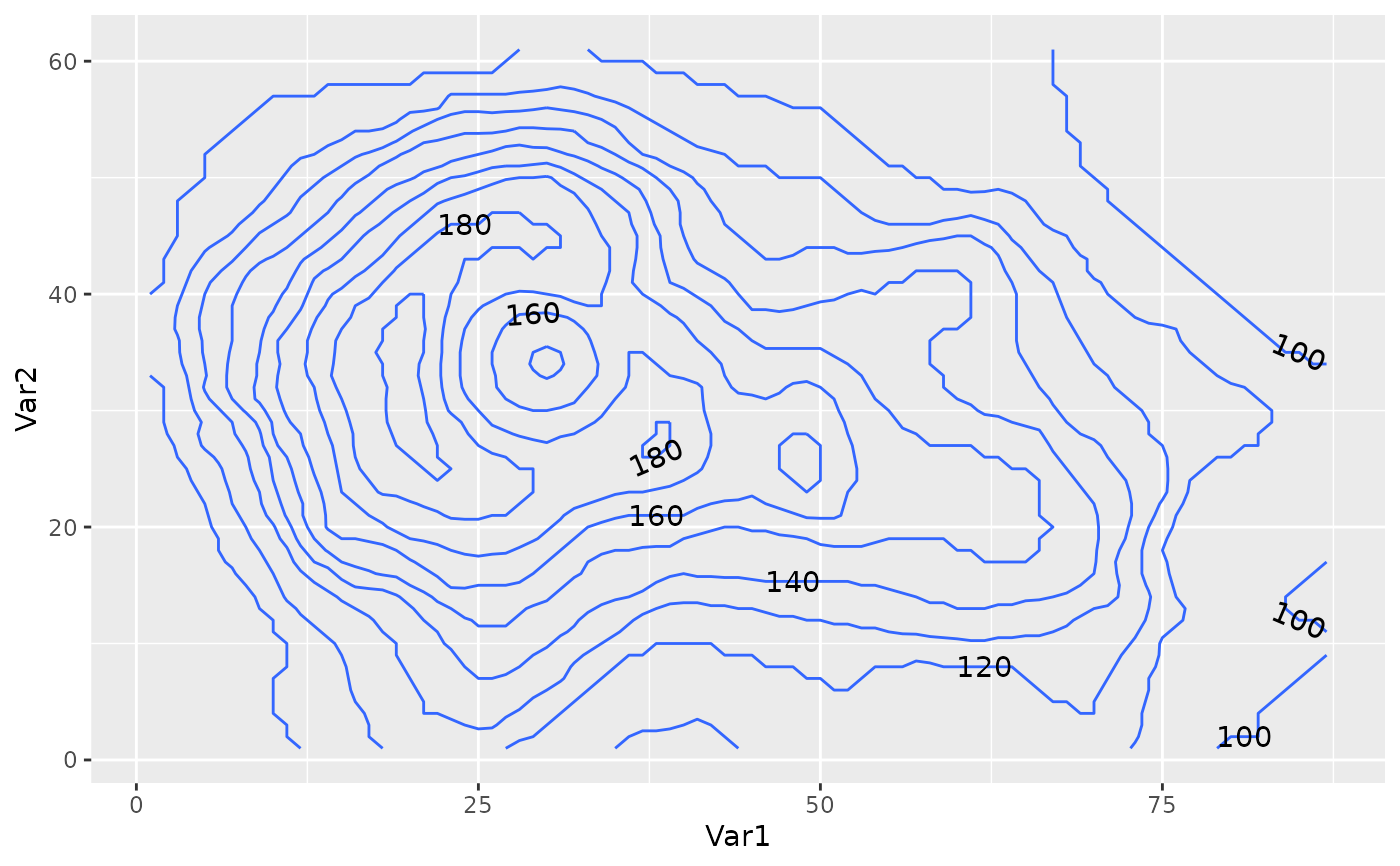g + geom_text_contour(aes(z = value), stroke = 0.2)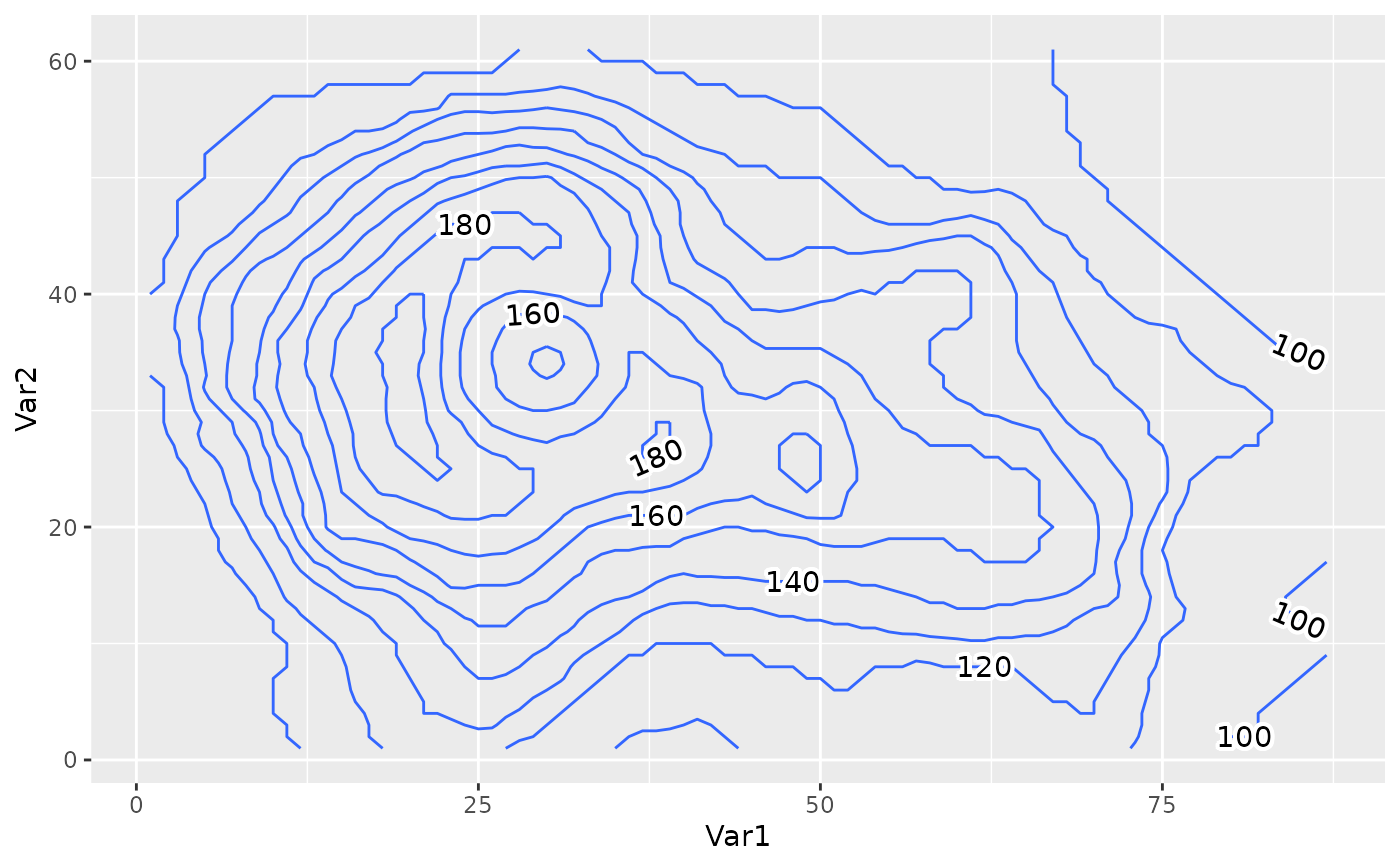g + geom_text_contour(aes(z = value), stroke = 0.2, stroke.colour = "red")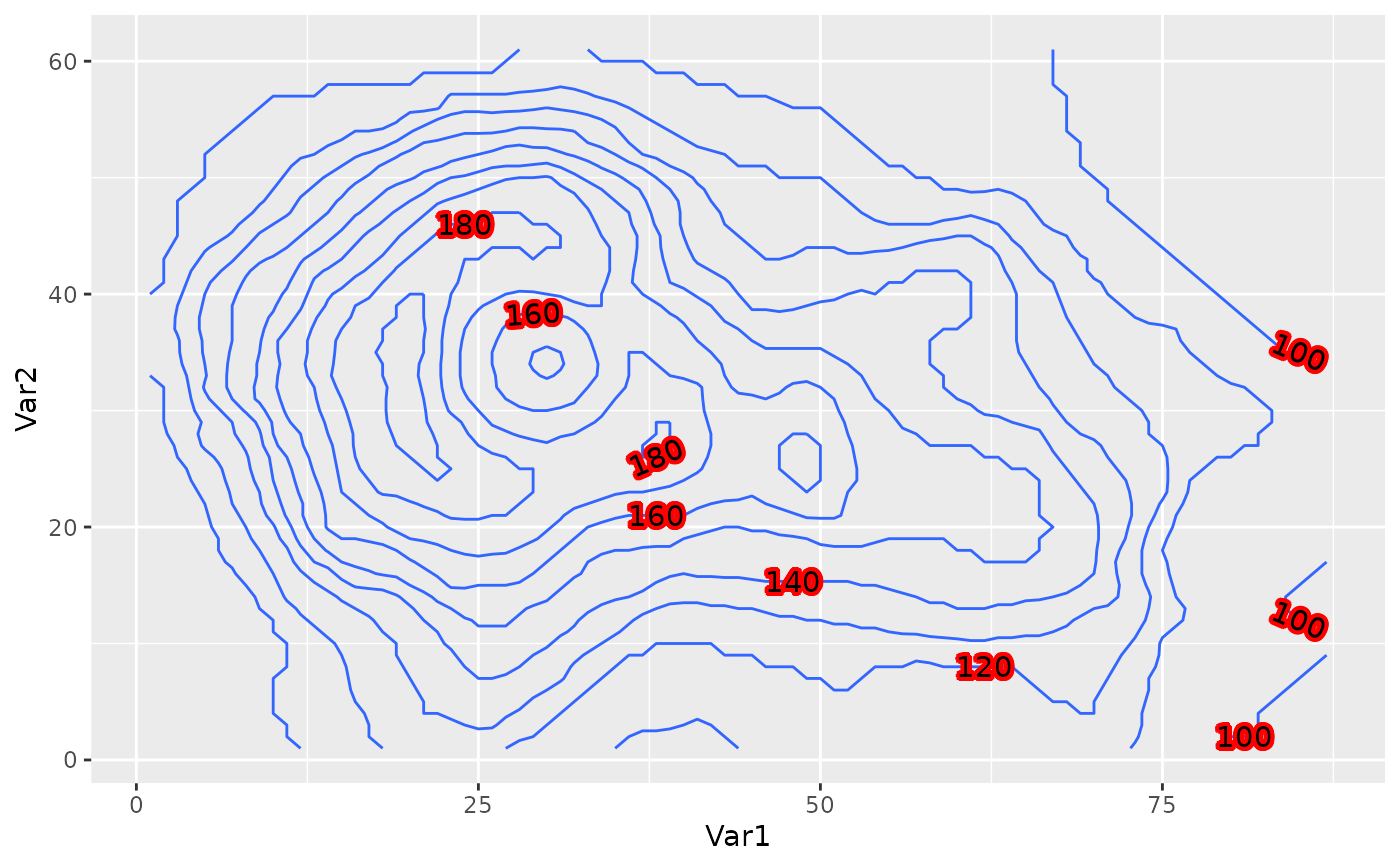g + geom_text_contour(aes(z = value, stroke.colour = after_stat(level)), stroke = 0.2) +
scale_colour_gradient(aesthetics = "stroke.colour", guide = "none")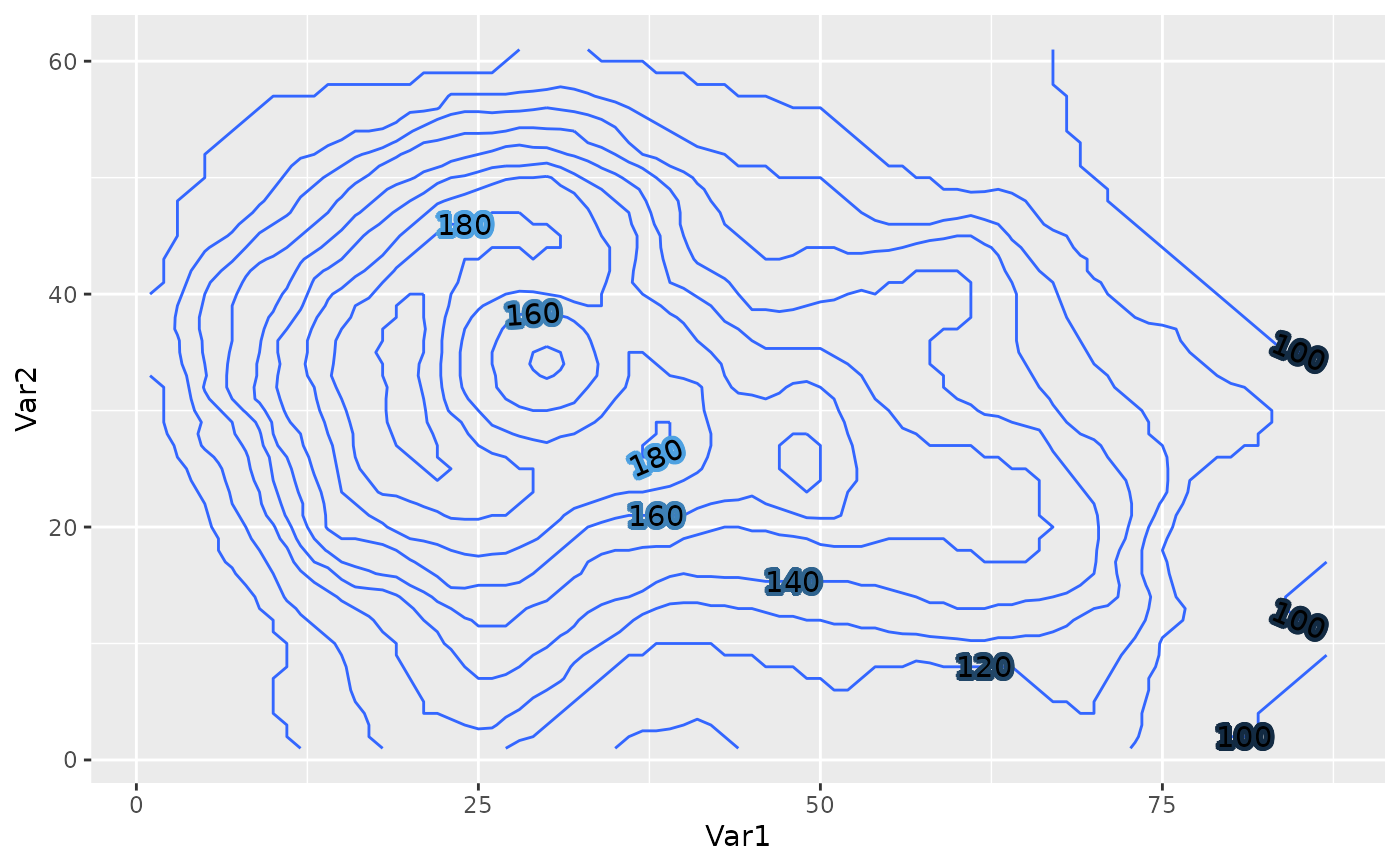g + geom_text_contour(aes(z = value), rotate = FALSE)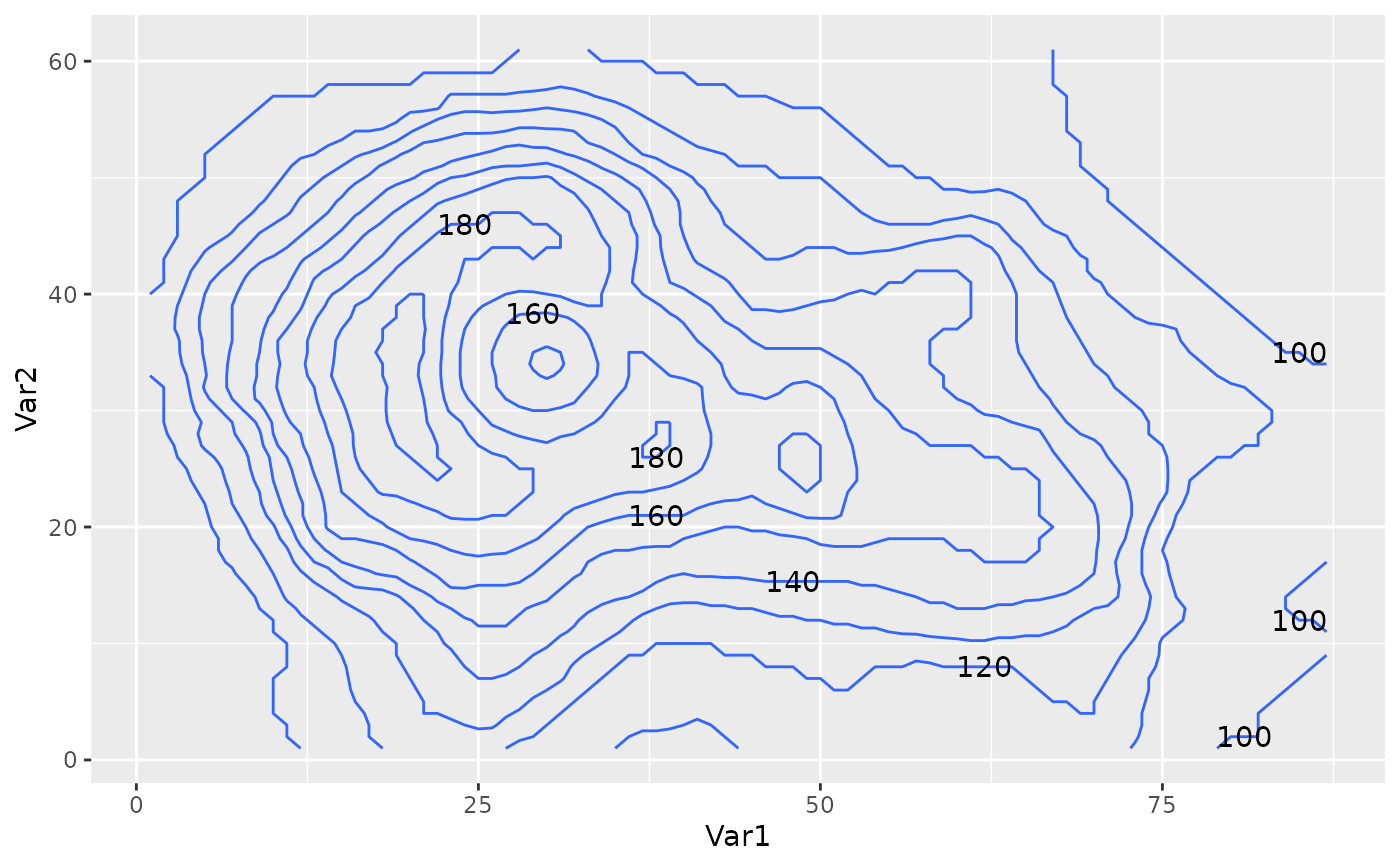g + geom_text_contour(aes(z = value),
label.placer = label_placer_random())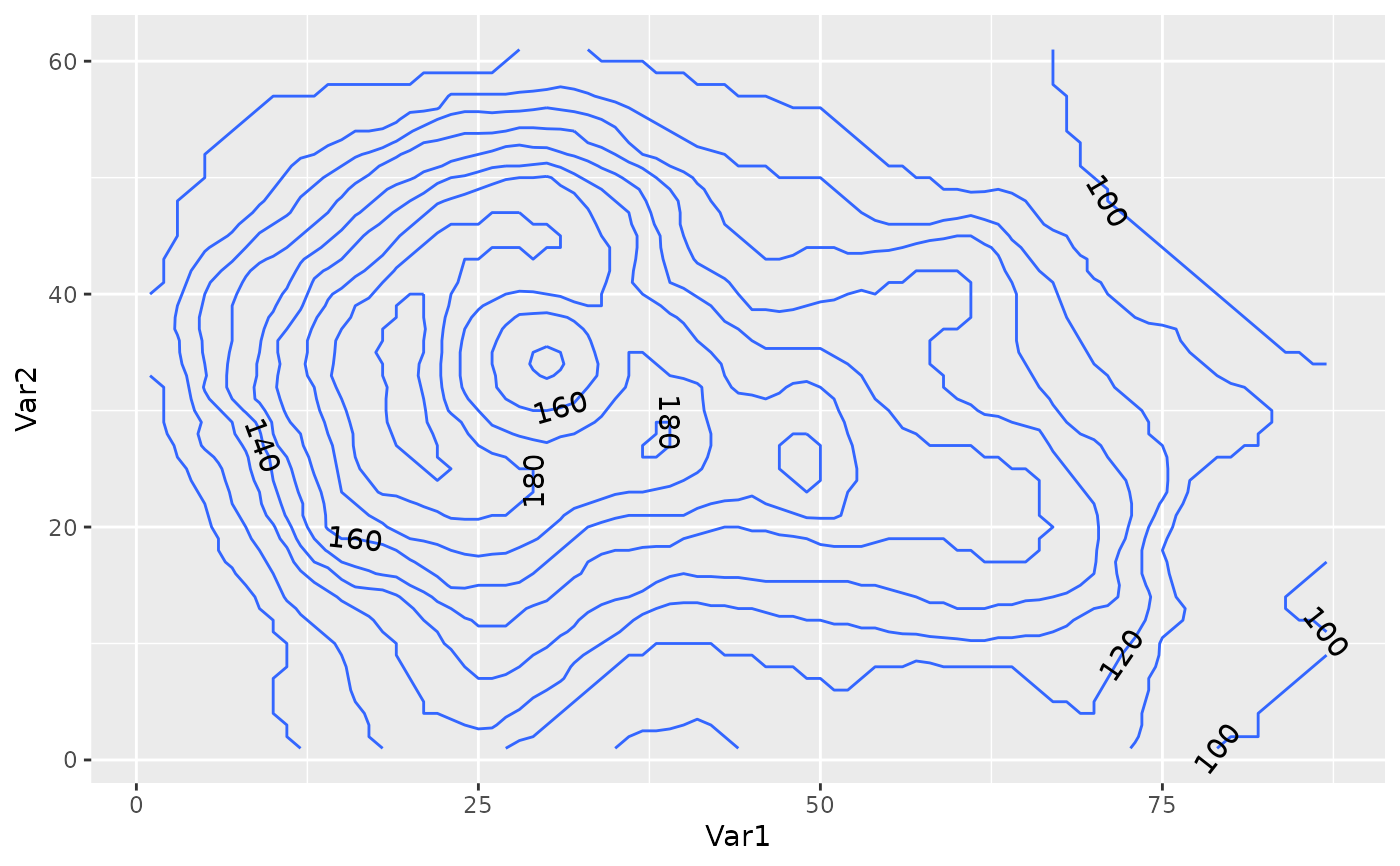g + geom_text_contour(aes(z = value),
label.placer = label_placer_n(3))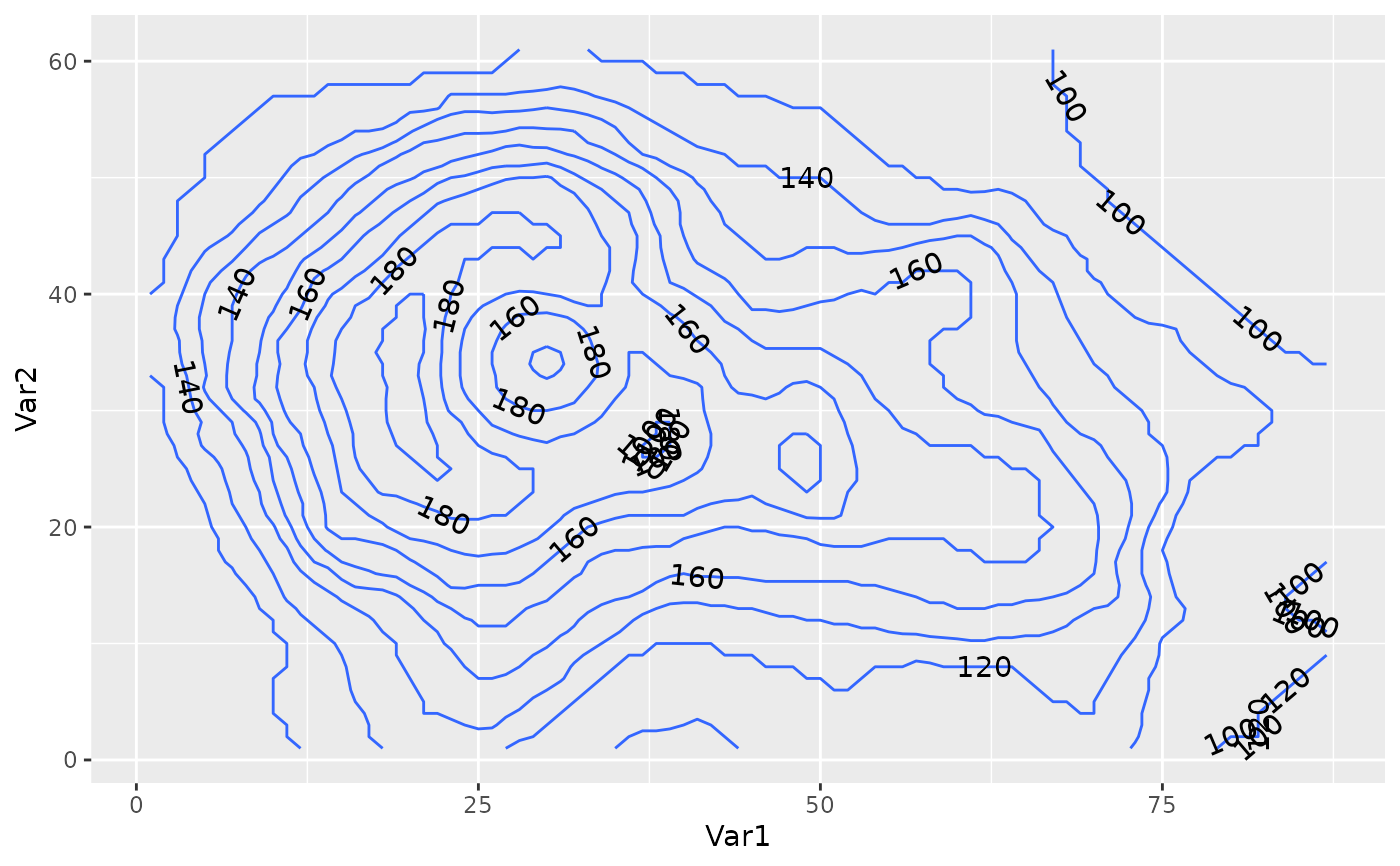g + geom_text_contour(aes(z = value),
label.placer = label_placer_flattest())g + geom_text_contour(aes(z = value),
label.placer = label_placer_flattest(ref_angle = 90))